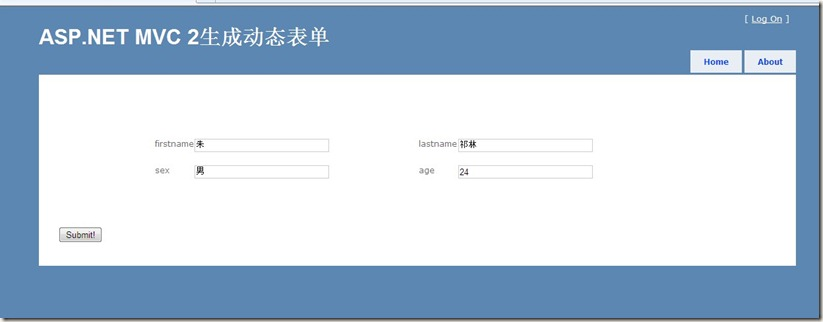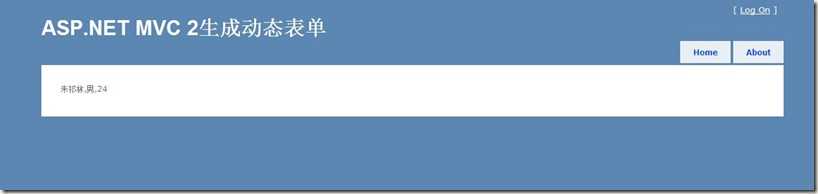# ASP.NET MVC 2生成动态表单的一种最简单的思路

2010-07-30 01:14 435 查看

```<?xml version="1.0" encoding="utf-8" ?>
<form name="form1">
<field type="text" name ="firstname" class ="textbox" left="300"  top="200">朱</field>
<field type="text" name ="lastname" class ="textbox" left="700"  top="200">祁林</field>
<field type="text" name ="sex" class ="textbox" left="300"  top="240">男</field>
<field type="text" name ="age" class ="textbox" left="700"  top="240">24</field>
</form>```

```[AcceptVerbs(HttpVerbs.Get)]
public ActionResult Index()
{
ViewData["xml"] = xml;
return View();
}

`下面，我定义两个字符串模板：`

```string label = " <div  style=\"left: {0}px; position: absolute; top: {1}px\">{2}</div>";
string input = "<input name=\"{0}\" type=\"{1}\"  class=\"{2}\"   style=\"left: {3}px; position: absolute; top: {4}px\" value=\"{5}\"  />";```

label用于显示文本信息，input用于显示表单上的value。下面通过循环产生html脚本。

```StringBuilder sb = new StringBuilder();
sb.Append("    <div style=\"height:200px\"> ");
foreach(XElement f in xml.Elements())
{
sb.Append(string.Format(label, int.Parse(f.Attribute("left").Value) - 60, f.Attribute("top").Value, f.Attribute("name").Value));
sb.Append(string.Format(input, f.Attribute("name").Value, f.Attribute("type").Value, f.Attribute("class").Value, f.Attribute("left").Value, f.Attribute("top").Value, f.Value));
}
sb.Append("</div > ");```

```<%@ Page Language="C#" MasterPageFile="~/Views/Shared/Site.Master" Inherits="System.Web.Mvc.ViewPage" %>

<asp:Content ID="Content1" ContentPlaceHolderID="TitleContent" runat="server">
</asp:Content>
<asp:Content ID="Content2" ContentPlaceHolderID="MainContent" runat="server">
<% XElement xml = (XElement)ViewData["xml"]; %>
<%Html.BeginForm(); %>```

```   <%string label = " <div  style=\"left: {0}px; position: absolute; top: {1}px\">{2}</div>";
string input = "<input name=\"{0}\" type=\"{1}\"  class=\"{2}\"   style=\"left: {3}px; position: absolute; top: {4}px\" value=\"{5}\"  />";StringBuilder sb = new StringBuilder();
sb.Append("    <div style=\"height:200px\"> ");
foreach(XElement f in xml.Elements())
{
sb.Append(string.Format(label, int.Parse(f.Attribute("left").Value) - 60, f.Attribute("top").Value, f.Attribute("name").Value));
sb.Append(string.Format(input, f.Attribute("name").Value, f.Attribute("type").Value, f.Attribute("class").Value, f.Attribute("left").Value, f.Attribute("top").Value, f.Value));
}
sb.Append("</div > ");Response.Write(sb.ToString());%><input type="submit" value="Submit!" /><%Html.EndForm(); %></asp:Content>```

1、显示XML表单：2、提交表单：